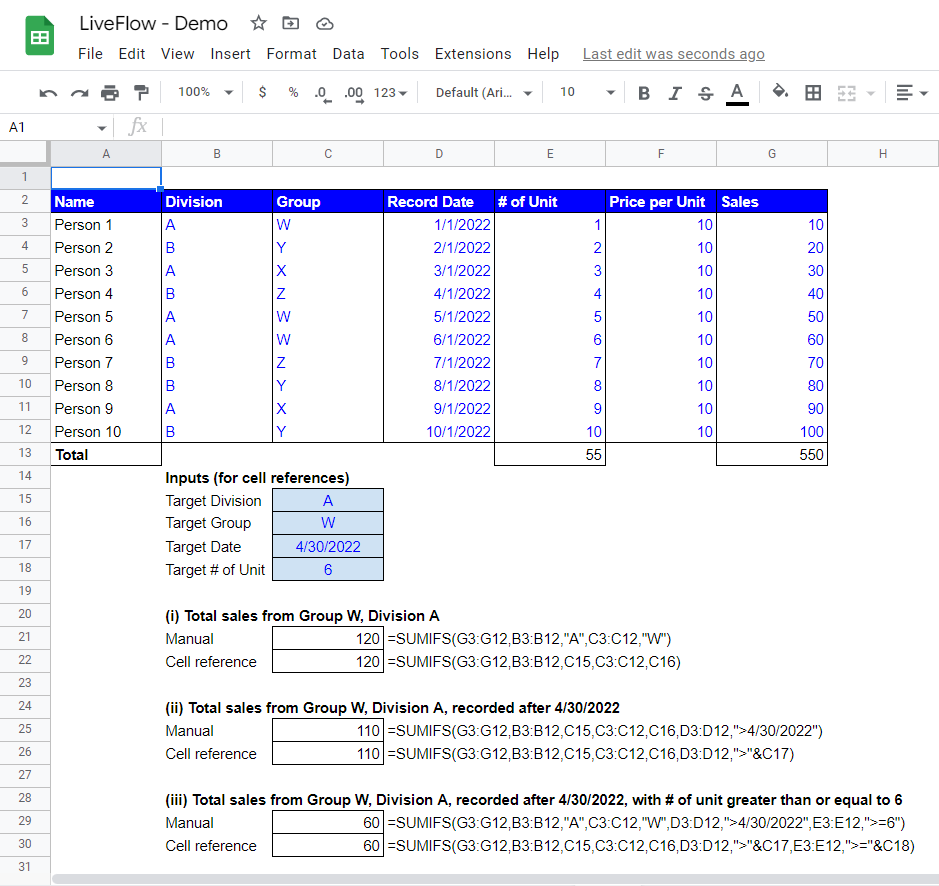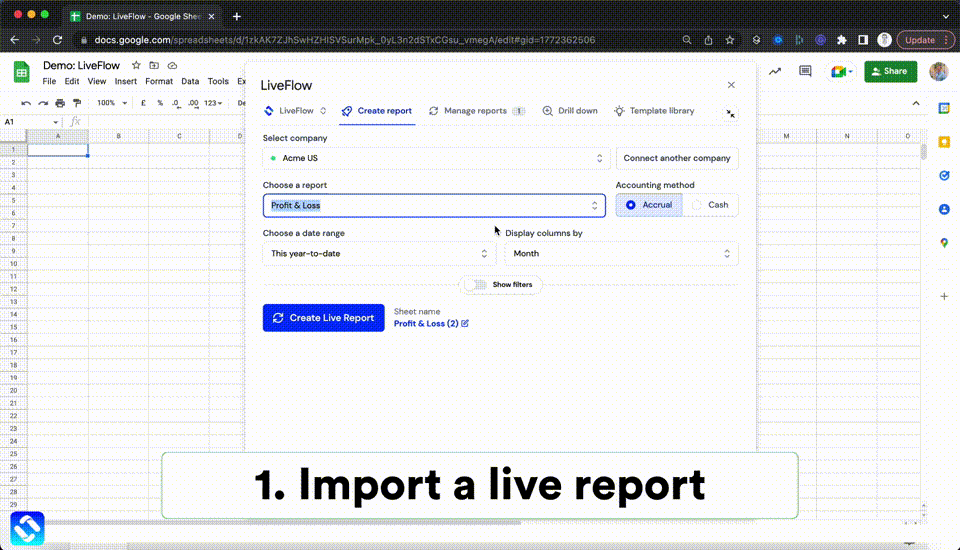# SUMIFS Function in Google Sheets: Explained

Learn how the SUMIFS function works in this article.

## How to use SUMIFS formula in Google Sheets

1. Type “=SUMIFS”* or go to the “Insert” ➝ “Function” ➝ “Math” ➝ “SUMIFS”.
2. Input a range** by selecting a field or type in a range** between the brackets***. In the SUMIFS formula, you can enter text, date, and numbers as criteria.
3. Press the Enter key on your keyboard.

*You don’t need to add quotation marks when you enter the formula name

**The number of cells in the selected ranges should be equal

***The SUMIFS shows a total number that meets all criteria input.

The following is a generic formula of the SUMIFS function.

``=SUMIFS(sum_range, criteria_range1, criterion1, [criteria_range2, …], [criterion2, …])``

Sum_range: This is a range that you want to add up

Criteria_range 1: This is a range where you want to check if each cell in this range matches a criterion

Criterion 1: This is a specific condition you would like to apply to the selected range (Criteria_range 1).

You will need to repeat inputting a pair of a criterion and a range until you incorporate all conditions.

Let’s see examples of how the function works. Imagine there’s a firm that wants to understand their sales performance by division from different standpoints.

In the examples, you will find two types of inputs in the formulas, “Manual” and “Cell references.” “Manual” means you type criteria manually in the formulas, and “Cell references” means that you refer to particular cells containing a specific number, date, or text. We highly recommend you take the latter approach as it is more flexible, and it helps you to reduce mistakes such as leaving an old criterion when you change the formula.

Total Sales from Group W, Division A

In the first example, the criteria are two inputs, “Division” and “Group”.

The “Inputs” group of cells defines our targets such as Group and Division.

The formula in C17 calculates how many sales Group W from Division A made in total and gives us the result we’re looking for.

Total Sales from Group W, Division A, recorded after 4/30/2022

The second example adds one more criterion, “Recorded Date” so that the outcome are the sales for Group W, Division A, recorded after 4/30/2022.

Total Sales from Group W, Division A, recorded after 4/30/2022, with the number of units greater than or equal to 6

In the third example, the number of units - “# of Unit” - is also included on top of the existing three criteria.Examples of SUMIFS formulas with text, cell references, and dates

Regardless of which approach you want to take, bear the following rules in mind:

• Numbers and cell references are not needed to be input with quotation marks.
• Text, wildcard (signs such as “*,” “?,” and “~.”) and date need to be input with quotation marks such as “A” or “*” or “8/25/2022”
• Comparison operators such as “=,” “<,” and “>” with figures, text, and dates need to be enclosed by double quotes such as “<=100,” “<>apple,” and “>8/25/2022”
• Comparison operator(s) with cell reference(s) or another formula(s) should require (i) quotation marks to enclose comparison operators, and (ii) “&” (ampersand) between the operators and formula(s) like “>=”&A1 or “<”&TODAY().

## How do I SUMIFS multiple criteria in one column in Google Sheets?

For simplification, let’s assume the number of criteria is two.

When you want to incorporate more than one criteria in a column, you can use curly brackets “{}” and the SUMIFS formula looks like this; =SUMIFS(sum_range W, criteria_range X, {criterion Y, criterion Z}). This formula brings you the total value of cells that match the criteria of C or D.

Here is another way to do this, but this approach is a bit more burdensome as you need to use SUMIFS functions as many as the criteria. Again, let’s assume you want to apply two standards to a column. You need to add up two results of SUMIFS functions and it should look like this; =SUMIFS(sum_range A, criteria_range B, criterion C)+SUMIFS(sum_range A, criteria_range B, criterion D); or you can use two SUMIF functions as well and it should look like this; =SUMIF(range B, criterion C, [sum_range A])+SUMIF(range B, criterion D, [sum_range A]). The difference between the two SUMIFS formulas (or SUMIF formulas) is the criterion.

## What is the difference between SUMIF and SUMIFS?

The SUMIF formula shows the total figures in cells which match a condition.

The SUMIFS function gives you the total figures in cells which meet multiple criteria. As you can see above, you can use both formulas, if you consider only one criterion. However, you should be aware that the order of necessary inputs in the formulas is different. Also, if you want to take multiple conditions into consideration, you need to use the SUMIFS function.

Move on to How to Use SUMIF formula in Google Sheets to learn the SUMIF function.

Are you learning this formula to process financial data, build up a financial model, or conduct financial analysis? If so, LiveFlow may help you to automate manual workflows and keep numbers updated in real timeAutomate any custom financial dashboard in Google Sheets with LiveFlow

Learn how to do this step-by-step in the video below 👇

## Do you need personal help?

Our team of real people are here to help you any time between 9am and 10pm GMT.Email us at: founders@liveflow.ioCall us at +1 (415) 650-1711